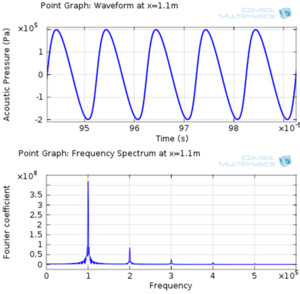# Application Gallery

#### Nonlinear Acoustics — Modeling of the 1D Westervelt Equation

Application ID: 12783

This model example shows how to model nonlinear propagation of 1D finite-amplitude Acoustic waves in fluids using Acoustics Module of COMSOL Multiphysics. The model is based on the 2nd order Westervelt equation.

The one dimensional nonlinear wave equation is solved in the time domain by adding the nonlinear term to the linear equation. The model does not include energy dissipation in order to compare the simulation results to the analytical solution. However, energy loss can be easily added in COMSOL. The analytical solution used for comparison is the Fubini solution which is valid for the region near the source.This model example illustrates applications of this type that would nominally be built using the following products: